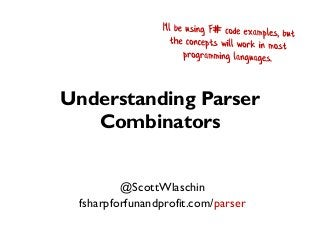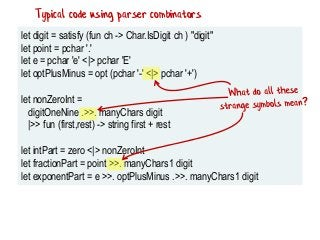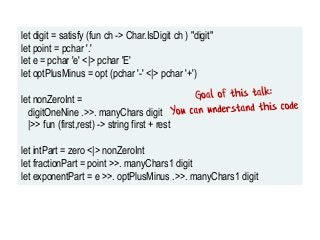Successfully reported this slideshow.

# Understanding parser combinators×

# Understanding parser combinators

Traditionally, writing parsers has been hard, involving arcane tools like Lex and Yacc. An alternative approach is to write a parser in your favourite programming language, using a "parser combinator" library and concepts no more complicated than regular expressions.

In this talk, we'll do a deep dive into parser combinators. We'll build a parser combinator library from scratch in F# using functional programming techniques, and then use it to implement a full featured JSON parser.

Code and video at https://fsharpforfunandprofit.com/parser/

Traditionally, writing parsers has been hard, involving arcane tools like Lex and Yacc. An alternative approach is to write a parser in your favourite programming language, using a "parser combinator" library and concepts no more complicated than regular expressions.

In this talk, we'll do a deep dive into parser combinators. We'll build a parser combinator library from scratch in F# using functional programming techniques, and then use it to implement a full featured JSON parser.

Code and video at https://fsharpforfunandprofit.com/parser/

### Understanding parser combinators

1. 1. Understanding Parser Combinators @ScottWlaschin fsharpforfunandprofit.com/parser
2. 2. let digit = satisfy (fun ch -> Char.IsDigit ch ) "digit" let point = pchar '.' let e = pchar 'e' <|> pchar 'E' let optPlusMinus = opt (pchar '-' <|> pchar '+') let nonZeroInt = digitOneNine .>>. manyChars digit |>> fun (first,rest) -> string first + rest let intPart = zero <|> nonZeroInt let fractionPart = point >>. manyChars1 digit let exponentPart = e >>. optPlusMinus .>>. manyChars1 digit Typical code using parser combinators
3. 3. let digit = satisfy (fun ch -> Char.IsDigit ch ) "digit" let point = pchar '.' let e = pchar 'e' <|> pchar 'E' let optPlusMinus = opt (pchar '-' <|> pchar '+') let nonZeroInt = digitOneNine .>>. manyChars digit |>> fun (first,rest) -> string first + rest let intPart = zero <|> nonZeroInt let fractionPart = point >>. manyChars1 digit let exponentPart = e >>. optPlusMinus .>>. manyChars1 digit
4. 4. Overview 1. What is a parser combinator library? 2. The foundation: a simple parser 3. Three basic parser combinators 4. Building combinators from other combinators 5. Improving the error messages 6. Building a JSON parser
5. 5. Part 1 What is a parser combinator library?
6. 6. Something to match Parser<something> Create step in parsing recipe Creating a parsing recipe A “Parser-making" function This is a recipe to make something, not the thing itself
7. 7. Parser<thingC> Combining parsing recipes A recipe to make a more complicated thing Parser<thingA> Parser<thingB> combined with A "combinator"
8. 8. Parser<something> Run Running a parsing recipe input Success or Failure
9. 9. Why parser combinators? • Written in your favorite programming language • No preprocessing needed – Lexing, parsing, AST transform all in one. – REPL-friendly • Easy to create little DSLs – Google "fogcreek fparsec" • Fun way of understanding functional composition
10. 10. Part 2: A simple parser
11. 11. Version 1 – parse the character 'A' input pcharA remaining input true/false
12. 12. Version 1 – parse the character 'A' input pcharA remaining input true/false
13. 13. let pcharA input = if String.IsNullOrEmpty(input) then (false,"") else if input. = 'A' then let remaining = input.[1..] (true,remaining) else (false,input)
14. 14. Version 2 – parse any character matched char input pchar remaining input charToMatch failure message
15. 15. let pchar (charToMatch,input) = if String.IsNullOrEmpty(input) then "No more input" else let first = input. if first = charToMatch then let remaining = input.[1..] (charToMatch,remaining) else sprintf "Expecting '%c'. Got '%c'" charToMatch first
16. 16. Fix – create a choice type to capture either case Success: matched char input pchar Success: remaining input charToMatch Failure: message type Result<'a> = | Success of 'a | Failure of string
17. 17. Fix – create a choice type to capture either case Success: matched char input pchar Success: remaining input charToMatch Failure: message type Result<'a> = | Success of 'a | Failure of string
18. 18. Fix – create a choice type to capture either case Success: matched char input pchar Success: remaining input charToMatch Failure: message type Result<'a> = | Success of 'a | Failure of string
19. 19. let pchar (charToMatch,input) = if String.IsNullOrEmpty(input) then Failure "No more input" else let first = input. if first = charToMatch then let remaining = input.[1..] Success (charToMatch,remaining) else let msg = sprintf "Expecting '%c'. Got '%c'" charToMatch firs Failure msg
20. 20. Version 3 – returning a function Success: matched char input pchar Success: remaining input charToMatch Failure: message
21. 21. Version 3 – returning a function Success: matched char input pchar Success: remaining input charToMatch Failure: message
22. 22. Version 3 – returning a function input pchar charToMatch
23. 23. Version 3 – returning a function charToMatch pchar
24. 24. Version 3 – returning a function charToMatch pchar
25. 25. Version 4 – wrapping the function in a type charToMatch pchar Parser<char>
26. 26. Version 4 – wrapping the function in a type charToMatch pchar Parser<char> type Parser<'a> = Parser of (string -> Result<'a * string>) A function that takes a string and returns a Result
27. 27. Version 4 – wrapping the function in a type charToMatch pchar Parser<char> type Parser<'a> = Parser of (string -> Result<'a * string>) Wrapper
28. 28. Creating parsing recipes
29. 29. charToMatch input Parser<char> A parsing recipe for a char
30. 30. Parser<something> Run Running a parsing recipe input Success, or Failure
31. 31. Running a parsing recipe input Parser<something> Parser<something> Run input Success, or Failure
32. 32. let run parser input = // unwrap parser to get inner function let (Parser innerFn) = parser // call inner function with input innerFn input
33. 33. Enough talk, show me some code
34. 34. Part 3: Three basic combinators
35. 35. What is a combinator? • A “combinator” library is a library designed around combining things to get more complex values of the same type. • integer + integer = integer • list @ list = list // @ is list concat • Parser ?? Parser = Parser
36. 36. Basic parser combinators • Parser andThen Parser => Parser • Parser orElse Parser => Parser • Parser map (transformer) => Parser
37. 37. AndThen parser combinator • Run the first parser. – If there is a failure, return. • Otherwise, run the second parser with the remaining input. – If there is a failure, return. • If both parsers succeed, return a pair (tuple) that contains both parsed values.
38. 38. let andThen parser1 parser2 = let innerFn input = // run parser1 with the input let result1 = run parser1 input // test the 1st parse result for Failure/Success match result1 with | Failure err -> Failure err // return error from parser1 | Success (value1,remaining1) -> // run parser2 with the remaining input (continued on next slide..)
39. 39. let andThen parser1 parser2 = [...snip...] let result2 = run parser2 remaining1 // test the 2nd parse result for Failure/Success match result2 with | Failure err -> Failure err // return error from parser2 | Success (value2,remaining2) -> let combinedValue = (value1,value2) Success (combinedValue,remaining2) // return the inner function Parser innerFn
40. 40. OrElse parser combinator • Run the first parser. • On success, return the parsed value, along with the remaining input. • Otherwise, on failure, run the second parser with the original input... • ...and in this case, return the result (success or failure) from the second parser.
41. 41. let orElse parser1 parser2 = let innerFn input = // run parser1 with the input let result1 = run parser1 input // test the result for Failure/Success match result1 with | Success result -> // if success, return the original result result1 | Failure err -> // if failed, run parser2 with the input (continued on next slide..)
42. 42. let orElse parser1 parser2 = [...snip...] | Failure err -> // if failed, run parser2 with the input let result2 = run parser2 input // return parser2's result result2 // return the inner function Parser innerFn
43. 43. Map parser combinator • Run the parser. • On success, transform the parsed value using the provided function. • Otherwise, return the failure
44. 44. let mapP f parser = let innerFn input = // run parser with the input let result = run parser input // test the result for Failure/Success match result with | Success (value,remaining) -> // if success, return the value transformed by f let newValue = f value Success (newValue, remaining) (continued on next slide..)
45. 45. let mapP f parser = [...snip...] | Failure err -> // if failed, return the error Failure err // return the inner function Parser innerFn
46. 46. Parser combinator operators pcharA .>>. pcharB // 'A' andThen 'B' pcharA <|> pcharB // 'A' orElse 'B' pcharA |>> (...) // map ch to something
47. 47. Demo
48. 48. Part 4: Building complex combinators from these basic ones
49. 49. [ 1; 2; 3] |> List.reduce (+) // 1 + 2 + 3 [ pcharA; pcharB; pcharC] |> List.reduce ( .>>. ) // pcharA .>>. pcharB .>>. pcharC [ pcharA; pcharB; pcharC] |> List.reduce ( <|> ) // pcharA <|> pcharB <|> pcharC Using reduce to combine parsers
50. 50. let choice listOfParsers = listOfParsers |> List.reduce ( <|> ) let anyOf listOfChars = listOfChars |> List.map pchar // convert char into Parser<char> |> choice // combine them all let parseLowercase = anyOf ['a'..'z'] let parseDigit = anyOf ['0'..'9'] Using reduce to combine parsers
51. 51. /// Convert a list of parsers into a Parser of list let sequence listOfParsers = let concatResults p1 p2 = // helper p1 .>>. p2 |>> (fun (list1,list2) -> list1 @ list2) listOfParsers // map each parser result to a list |> Seq.map (fun parser -> parser |>> List.singleton) // reduce by concatting the results of AndThen |> Seq.reduce concatResults Using reduce to combine parsers
52. 52. /// match a specific string let pstring str = str // map each char to a pchar |> Seq.map pchar // convert to Parser<char list> |> sequence // convert Parser<char list> to Parser<char array> |>> List.toArray // convert Parser<char array> to Parser<string> |>> String Using reduce to combine parsers
53. 53. Demo
54. 54. Yet more combinators
55. 55. “More than one” combinators let many p = ... // zero or more let many1 p = ... // one or more let opt p = ... // zero or one // example let whitespaceChar = anyOf [' '; 't'; 'n'] let whitespace = many1 whitespaceChar
56. 56. “Throwing away” combinators p1 .>> p2 // throw away right side p1 >>. p2 // throw away left side // keep only the inside value let between p1 p2 p3 = p1 >>. p2 .>> p3 // example let pdoublequote = pchar '"' let quotedInt = between pdoublequote pint pdoublequote
57. 57. “Separator” combinators let sepBy1 p sep = ... /// one or more p separated by sep let sepBy p sep = ... /// zero or more p separated by sep // example let comma = pchar ',' let digit = anyOf ['0'..'9'] let oneOrMoreDigitList = sepBy1 digit comma
58. 58. Demo
59. 59. Part 5: Improving the error messages
60. 60. input Parser<char> Named parsers Name: “Digit” Parsing Function:
61. 61. Named parsers let ( <?> ) = setLabel // infix version run parseDigit "ABC" // without the label // Error parsing "9" : Unexpected 'A' let parseDigit_WithLabel = anyOf ['0'..'9'] <?> "digit" run parseDigit_WithLabel "ABC" // with the label // Error parsing "digit" : Unexpected 'A'
62. 62. input Parser<char> Extra input context Input: * Stream of characters * Line, Column
63. 63. Extra input context run pint "-Z123" // Line:0 Col:1 Error parsing integer // -Z123 // ^Unexpected 'Z' run pfloat "-123Z45" // Line:0 Col:4 Error parsing float // -123Z45 // ^Unexpected 'Z'
64. 64. Part 6: Building a JSON Parser
65. 65. // A type that represents the previous diagram type JValue = | JString of string | JNumber of float | JObject of Map<string, JValue> | JArray of JValue list | JBool of bool | JNull
66. 66. Parsing JSON Null
67. 67. // new helper operator. let (>>%) p x = p |>> (fun _ -> x) // runs parser p, but ignores the result // Parse a "null" let jNull = pstring "null" >>% JNull // map to JNull <?> "null" // give it a label
68. 68. Parsing JSON Bool
69. 69. // Parse a boolean let jBool = let jtrue = pstring "true" >>% JBool true // map to JBool let jfalse = pstring "false" >>% JBool false // map to JBool // choose between true and false jtrue <|> jfalse <?> "bool" // give it a label
70. 70. Parsing a JSON String
71. 71. Call this "unescaped char"
72. 72. /// Parse an unescaped char let jUnescapedChar = let label = "char" satisfy (fun ch -> (ch <> '') && (ch <> '"') ) label
73. 73. Call this "escaped char"
74. 74. let jEscapedChar = [ // each item is (stringToMatch, resultChar) (""",'"') // quote ("",'') // reverse solidus ("/",'/') // solidus ("b",'b') // backspace ("f",'f') // formfeed ("n",'n') // newline ("r",'r') // cr ("t",'t') // tab ] // convert each pair into a parser |> List.map (fun (toMatch,result) -> pstring toMatch >>% result) // and combine them into one |> choice <?> "escaped char" // set label
75. 75. Call this "unicode char"
76. 76. "unescaped char" or "escaped char" or "unicode char"
77. 77. let quotedString = let quote = pchar '"' <?> "quote" let jchar = jUnescapedChar <|> jEscapedChar <|> jUnicodeChar // set up the main parser quote >>. manyChars jchar .>> quote let jString = // wrap the string in a JString quotedString |>> JString // convert to JString <?> "quoted string" // add label
78. 78. Parsing a JSON Number
79. 79. "int part" "sign part"
80. 80. let optSign = opt (pchar '-') let zero = pstring "0" let digitOneNine = satisfy (fun ch -> Char.IsDigit ch && ch <> '0') "1-9" let digit = satisfy (fun ch -> Char.IsDigit ch ) "digit" let nonZeroInt = digitOneNine .>>. manyChars digit |>> fun (first,rest) -> string first + rest // set up the integer part let intPart = zero <|> nonZeroInt
81. 81. "fraction part"
82. 82. // set up the fraction part let point = pchar '.' let fractionPart = point >>. manyChars1 digit
83. 83. "exponent part"
84. 84. // set up the exponent part let e = pchar 'e' <|> pchar 'E' let optPlusMinus = opt (pchar '-' <|> pchar '+') let exponentPart = e >>. optPlusMinus .>>. manyChars1 digit
85. 85. "exponent part" "int part" "fraction part""sign part"
86. 86. // set up the main JNumber parser optSign .>>. intPart .>>. opt fractionPart .>>. opt exponentPart |>> convertToJNumber // not shown <?> "number" // add label
87. 87. Parsing JSON Arrays and Objects
88. 88. Completing the JSON Parser
89. 89. // the final parser combines the others together let jValue = choice [ jNull jBool jNumber jString jArray jObject ]
90. 90. Demo: the JSON parser in action
91. 91. Summary • Treating a function like an object – Returning a function from a function – Wrapping a function in a type • Working with a "recipe" (aka "effect") – Combining recipes before running them. • The power of combinators – A few basic combinators: "andThen", "orElse", etc. – Complex parsers are built from smaller components. • Combinator libraries are small but powerful – Less than 500 lines for combinator library – Less than 300 lines for JSON parser itself
92. 92. Want more? • For a production-ready library for F#, search for "fparsec" • There are similar libraries for other languages
93. 93. Thanks! @ScottWlaschin fsharpforfunandprofit.com/parser Contact me Slides and video here Let us know if you need help with F#